# Hens and pigs

Hens and pigs have 46 feet in total. At least how much can heads have?

Result

n =  12

#### Solution:

$46=2s+4p \ \\ p=46/4=\dfrac{ 23 }{ 2 }=11.5 \ \\ \ \\ p_{1}=\lfloor p \rfloor=\lfloor 11.5 \rfloor=11 \ \\ s_{1}=(46 - 4 \cdot \ p_{1})/2=(46 - 4 \cdot \ 11)/2=1 \ \\ \ \\ n=s_{1}+p_{1}=1+11=12$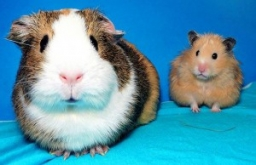Our examples were largely sent or created by pupils and students themselves. Therefore, we would be pleased if you could send us any errors you found, spelling mistakes, or rephasing the example. Thank you!

Leave us a comment of this math problem and its solution (i.e. if it is still somewhat unclear...):Be the first to comment!#### You need to know the following knowledge to solve this word math problem:

We encourage you to watch this tutorial video on this math problem:

## Next similar math problems:

1. Florist's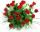The florist got 72 white and 90 red roses. How many bouquets can bind from all these roses when each bouquets should have the same number of white and red roses?
2. The percent 2The percent return rate of a growth fund, income fund, and money market are 10%, 7%, and 5% respectively. Suppose you have 3200 to invest and you want to put twice as much in the growth fund as in the money market to maximize your return. How should you i
3. Secret treasureScouts have a tent in the shape of a regular quadrilateral pyramid with a side of the base 4 m and a height of 3 m. Determine the radius r (and height h) of the container so that they can hide the largest possible treasure.
4. ClassmatesRoman is ranked 12th highest and eleventh lowest pupil. How many classmates does Roman have?
5. TV competitionIn the competition, 10 contestants answer five questions, one question per round. Anyone who answers correctly will receive as many points as the number of competitors answered incorrectly in that round. One of the contestants after the contest said: We
6. Endless lego setThe endless lego set contains only 6, 9, 20 kilograms blocks that can no longer be polished or broken. The workers took them to the gym and immediately started building different buildings. And of course, they wrote down how much the building weighed. The
7. Cylindrical containerAn open-topped cylindrical container has a volume of V = 3140 cm3. Find the cylinder dimensions (radius of base r, height v) so that the least material is needed to form the container.
8. Z9–I–1In all nine fields of given shape to be filled natural numbers so that: • each of the numbers 2, 4, 6 and 8 is used at least once, • four of the inner square boxes containing the products of the numbers of adjacent cells of the outer square, • in the ci
9. Ten boys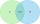Ten boys chose to go to the supermarket. Six boys bought gum and nine boys bought a lollipop. How many boys bought gum and a lollipop?
10. Test scoresJo's test scores on the first four 100 point exams are as follows: 96,90,76, and 88. If all exams are worth the same percent, what is the minimum test score necessary on his last exam to earn an A grade in the class (90% or better)?
11. Minimum surfaceFind the length, breadth, and height of the cuboid shaped box with a minimum surface area, into which 50 cuboid shaped blocks, each with length, breadth and height equal to 4 cm, 3 cm and 2 cm respectively can be packed.
12. Curve and lineThe equation of a curve C is y=2x² -8x+9 and the equation of a line L is x+ y=3 (1) Find the x co-ordinates of the points of intersection of L and C. (2) Show that one of these points is also the stationary point of C?4 m long ladder touches the cube 1mx1m at the wall. How high reach on the wall?There were 16 passenger cars in the parking. It was the 10 blue and 10 Skoda cars. How many are blue Skoda cars in the parking?Hard rectangular paper has dimensions of 60 cm and 28 cm. The corners are cut off equal squares and the residue was bent to form an open box. How long must be side of the squares to be the largest volume of the box?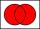In the class was 12 students. Nine students wearing trousers and turtleneck eight. How many students worn trousers with a turtleneck?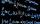Find a positive number that the sum of the number and its inverted value was minimal.